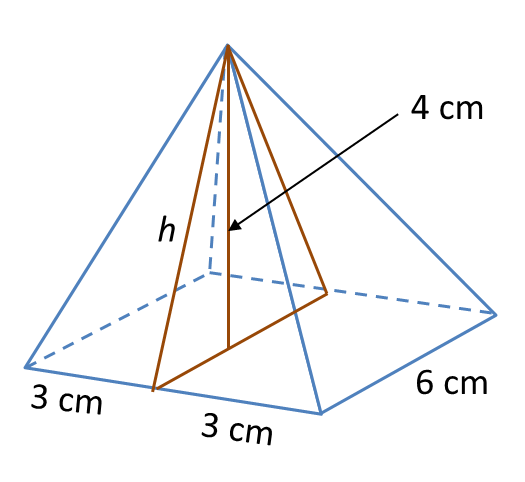12.2.2 Solid Geometry (II), PT3 Focus Practice

12.2.2 Solid Geometry (II), PT3 Focus Practice

Question 5:
Sphere below has a surface area of 221.76 cm2.$\left(\pi =\frac{22}{7}\right)$

Solution:
Surface area of sphere = 4πr2
$\begin{array}{l}4\pi {r}^{2}=221.76\\ 4×\frac{22}{7}×{r}^{2}=221.76\\ {r}^{2}=\frac{221.76×7}{4×22}\\ {r}^{2}=17.64\\ r=\sqrt{17.64}\\ r=4.2\text{cm}\end{array}$

Question 6:
Diagram below shows a right pyramid with a square base.Given the height of the pyramid is 4 cm.
Calculate the total surface area, in cm2, of the right pyramid.

Solution:h2 = 32 + 42
= 9 + 16
= 25
h = √25   = 5 cm2

Total surface area of the right pyramid
= Base area + 4 (Area of triangle)
= (6 × 6) + 4 × 4 (½ × 6 × 5)
= 36 + 60
= 96 cm2

Question 7:
Diagram below shows a prism.Draw to full scale, the net of the prism on the grid in the answer space. The grid has equal squares with sides of 1 unit.

Solution: